Courses

# Test: Kirchhoff’s Laws & Network Solutions

## 10 Questions MCQ Test Basic Electrical Technology | Test: Kirchhoff’s Laws & Network Solutions

Description
This mock test of Test: Kirchhoff’s Laws & Network Solutions for Electrical Engineering (EE) helps you for every Electrical Engineering (EE) entrance exam. This contains 10 Multiple Choice Questions for Electrical Engineering (EE) Test: Kirchhoff’s Laws & Network Solutions (mcq) to study with solutions a complete question bank. The solved questions answers in this Test: Kirchhoff’s Laws & Network Solutions quiz give you a good mix of easy questions and tough questions. Electrical Engineering (EE) students definitely take this Test: Kirchhoff’s Laws & Network Solutions exercise for a better result in the exam. You can find other Test: Kirchhoff’s Laws & Network Solutions extra questions, long questions & short questions for Electrical Engineering (EE) on EduRev as well by searching above.
QUESTION: 1

### Find the value of I1, I2 and I3.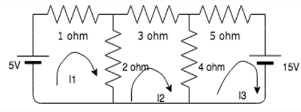Solution:

Using the matrix method:
Matrix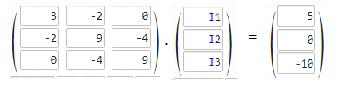Solving this matrix equation, we get I1=1.5A,

I2=-0.188A

I3=-1.19A.

QUESTION: 2

### Find the value of V, if the value of I3= 0A.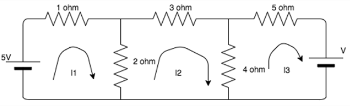Solution:

5-3I1+2I2=0, 9I2-2I1=0, -4I2+V=0
On solving,V=1.739V.

QUESTION: 3

### Find the value of R if the power in the circuit is 1000W.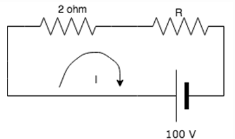Solution:

To find the value of I:
VI=P =>100I=1000 => I=10A.
Voltage across the 2 ohm resistor= 20V.
Voltage across the R resistor= 100-20= 80V.
R=V/I => R=80/10= 8ohm.

QUESTION: 4

Find the current in the 4 ohm resistor.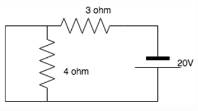Solution:

The 4 ohm resistor gets shorted since current always prefers the low resistance path. All the current flows to the branch which is connected in parallel to the 4 ohm branch, hence no current flows in the 4 ohm resistance.

QUESTION: 5

Nodal analysis is generally used to determine_______

Solution:

Nodal analysis uses Kirchhoff’s Current Law to find all the node voltages. Hence it is a method used to determine voltage.

QUESTION: 6

Mesh analysis is generally used to determine_________

Solution:

Mesh analysis uses Kirchhoff’s Voltage Law to find all the mesh currents. Hence it is a method used to determine current.

QUESTION: 7

What is the current in the circuit?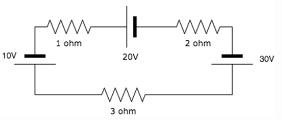Solution:

If we move in the clockwise direction, we get the total voltage to be equal to: -10-20+30= 0V. Since I=V/R= 0/4=0, I=0A.

QUESTION: 8

Does the 15A source have any effect on the circuit?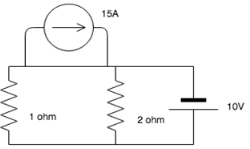Solution:

The 15A current source has a lower resistance path associated with it and hence it keeps moving in that particular loop. It does not leave that loop and enter the circuit, hence the circuit is not affected by it.

QUESTION: 9

KVL is associated with____________

Solution:

KVL employs mesh analysis to find the different mesh currents by finding the IR products in each mesh.

QUESTION: 10

Kirchhoff's laws are valid for

Solution:

KCL employs nodal analysis to find the different node voltages by finding the value if current in each branch.